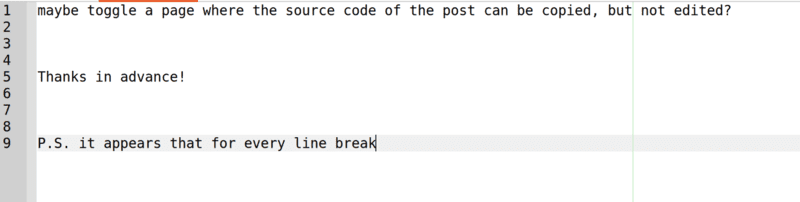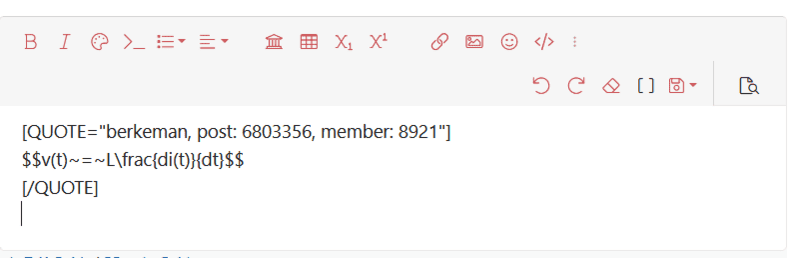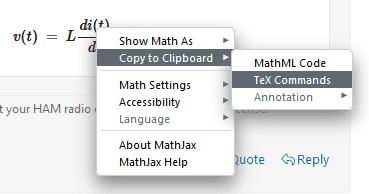# Can we see the latex source code for each post?

• Suggestion
yucheng
I was hoping to copy whole posts, along with the LATEX code (not the rendered equations). One way I did it in the Stackexchange network is to toggle the edit page and copy the source code there.

Apparently posts by others in PF, even your own posts after some time, cannot be edited. It would be great if we could at least copy the source code, perhaps by toggling a page where the source code of the post can be copied, but not edited?

P.S. it appears that for every line break copied from the text in the PF post editor (press the enter key), it is multiplied by two when pasted into, for instance Geany. Why is there such a behaviour?•Passerby

Mentor
If you have the BB toggle set so the icons are red and you "Reply" to the post, do you see the raw LaTeX? I usually do...

•yucheng
yucheng
Yes I just realized that haha quick work around... Thanks!

•berkeman
Mentor
$$v(t)~=~L\frac{di(t)}{dt}$$

Mentor$$v(t)~=~L\frac{di(t)}{dt}$$

•yucheng
Gold Member
By the way, if you just want to copy the LaTeX for a single equation rather than an entire post, just right-click on the rendered equation and select either "Show Math As >" or "Copy to Clipboard >" as appropriate.••berkeman, Office_Shredder and Wrichik Basu
Gold Member
2022 Award
By the way, if you just want to copy the LaTeX for a single equation rather than an entire post, just right-click on the rendered equation and select either "Show Math As >" or "Copy to Clipboard >" as appropriate.
That's a good way, unless the ##\LaTeX## code has some “hidden” code that MathJax executes but doesn't display. ##\require{physics} \require{newcommand} \def\jjmm{{j_1 j_2; m_1 m_2}} \def\jjjm{{j_1 j_2; j m}}##

But once you see the code, you will probably be able to guess that there is some more code hidden elsewhere in the post.
$$\begin{equation} \ip{\jjmm}{\jjjm} = (-1)^{j_1 - j_2 + m} \sqrt{2j + 1} \begin{pmatrix} j_1 & j_2 & j \\ m_1 & m_2 & -m \end{pmatrix}. \end{equation}$$

•DrGreg
Mentor
That's a good way, unless the ##\LaTeX## code has some “hidden” code that MathJax executes but doesn't display. ##\require{physics} \require{newcommand} \def\jjmm{{j_1 j_2; m_1 m_2}} \def\jjjm{{j_1 j_2; j m}}##

But once you see the code, you will probably be able to guess that there is some more code hidden elsewhere in the post.
$$\ip{\jjmm}{\jjjm} = ...$$
That's technically possible, but almost never used in actual posts.

yucheng
That's technically possible, but almost never used in actual posts.
Now I see the hidden code >u<